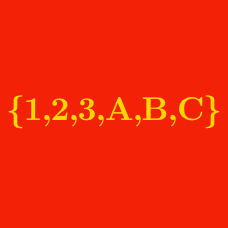Probability

# Sets - Classical Sets

If $\mathbb{R}$ denotes the set of real numbers, and $S$ is the set

$S = \{ 7, 1+\sqrt{2}, \pi, \sqrt{-11}, -11, 0, (-6)^3, -\sqrt{2} \} ,$

how many elements does $\mathbb{R} \cap S$ have?

Details and assumptions

You may choose to read the summary page Set Notation.

If $\mathbb{Q}$ denotes the set of rational numbers, and $S$ is the set

$S = \{ 9, 1+\sqrt{2}, \pi, \frac{5}{19}, \sqrt{-14}, -9, 0, (-6)^3, -\sqrt{2} \} ,$

how many elements does $\mathbb{Q} \cap S$ have?

Details and assumptions

You may choose to read the summary page Set Notation.

How many positive integers are there in the set

$\{ x \mid -14 \le x < 66\}?$

How many negative integers are there in the set

$\{ x \mid -15 \le x < 37\}?$

How many elements are in the set $\{10, 12, 14, \ldots, 48, 50\}$?

×